#### Manipulation graph

The manipulation planning problem generally can be solved by forming a manipulation graph,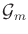[16,17]. Let a connected component of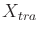refer to any connected component of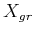that is lifted into the state space by ignoring the mode. There are two copies of the connected component of, one for each mode. For each connected component of, a vertex exists in. An edge is defined for each transfer path or transit path that connects two connected components of. The general approach to manipulation planning then is as follows:

1. Compute the connected components ofto yield the vertices of.
2. Compute the edges ofby applying ordinary motion planning methods to each pair of vertices of.
3. Apply motion planning methods to connect the initial and goal states to every possible vertex ofthat can be reached without a mode transition.
4. Searchfor a path that connects the initial and goal states. If one exists, then extract the corresponding solution as a sequence of transit and transfer paths (this yields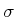, the actions that cause the required mode changes).
This can be considered as an example of hierarchical planning, as described in Section 1.4.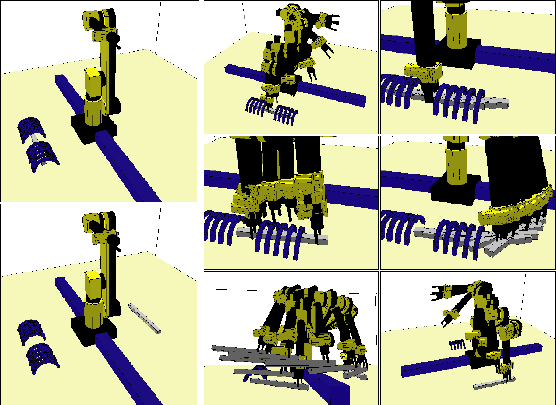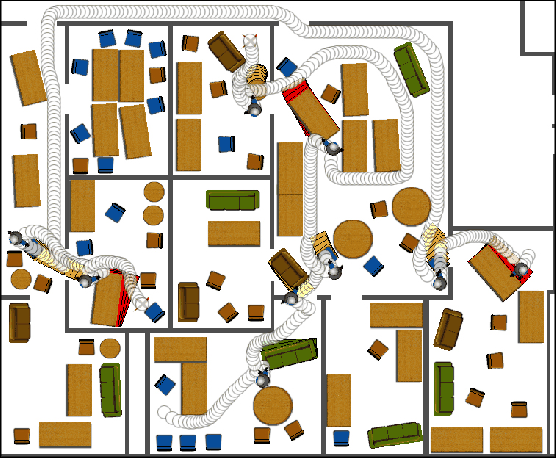Steven M LaValle 2020-08-14